# Bloch's Theorem with proof - Engineering Physics

Bloch Theorem

The band theory of solid assume that electron move in a periodic potential of the period ‘a’ (lattice constant).

i.e

The Schrodinger's equation for free-electron moving in a constant potential V0 is given as

So the Schrodinger's equation for an electron moving in periodic potential V(x) is written as

The solution of Eq. (3) are of the type

is known as Bloch function and which is again periodic with the same periodicity of the lattice.

i.e.

Thus Bloch Theorem is a mathematical statement regarding the form of the one-electron wave function for a perfectly periodic potential.

Proof -

We know that Schrodinger wave eq. (3) is a second-order differential eq. and hence there exist only two real independent solutions for this equation.

Say f(x) and g(x)  any other solution will be simply a linear combination of independent solutions.

Hence f(x+a) and g(x+a) are to be solutions of the eq. (3), there can be written as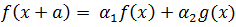...(7)

Now eq. (13) have non-vanishing solutions for A and B if and only if the determinant of the coefficient is zero, i.e.,

in eq. (15) and this result is proved below this topic.

Let us consider two cases for the energy ranges.

In this case the two roots will be the complex conjugate of each other, we can write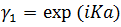and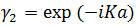Where K is real and the corresponding wave functions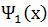can be written as

i.e., in general
Case 2. For energy range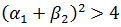In this case the roots are real and will be reciprocals of each other these correspond to wave functions of the type

And

is real. These are not acceptable wave functions since these are not bounded and approaches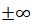when approaches infinity. Thus we find that the energy spectrum of an electron in a periodic potential consists of allowed and forbidden energy regions as bands. This will further be discussed in Kroning-Penney Model.

-----------------------------

As f(x) and g(x) are solutions of Schrodinger eq. (3), we have

Multiply f eq. by g and eq. g by f and then substracting, we get

Or

i.e the wronskian

Now from eq. (7) and (8), we may write